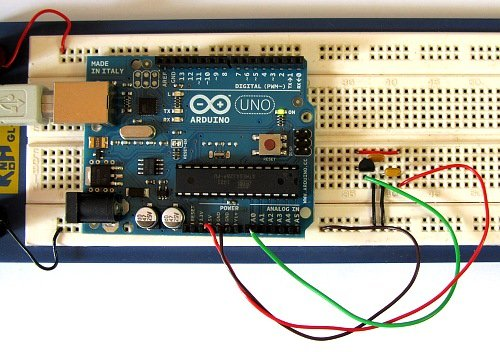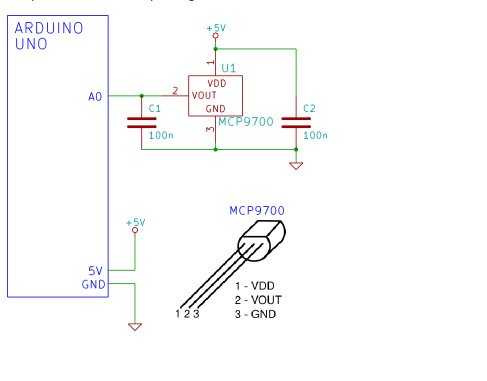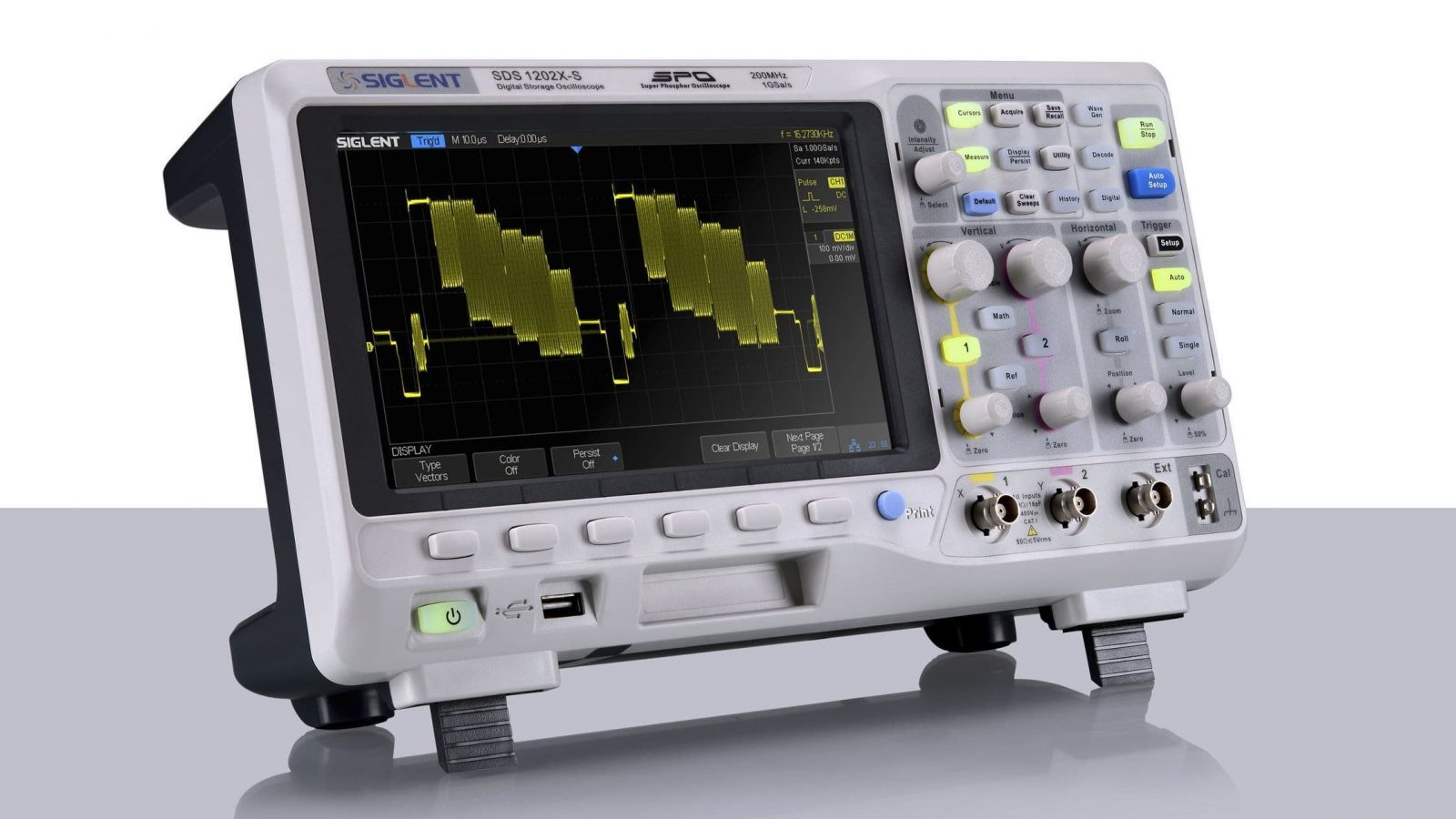# Arduino Serial Thermometer

The Arduino reads temperature from a MCP9700 temperature sensor IC and displays the temperature in the Arduino IDE serial monitor window.Also see the Arduino LCD thermometer tutorial (tutorial 14).

## Prerequisites

Complete Tutorial 9: Using the Arduino Serial Port before attempting this tutorial.

## Components

Besides an Arduino Uno board, USB cable, wire links and a breadboard, you will need:

QTY PART DESIGNATOR NOTES TYPE
2 100n C1, C2 Non-polarized Capacitor
1 MCP9700 U1 Linear Active Thermistor IC Semiconductor

## Circuit DiagramThe schematic for the Arduino serial thermometer and pinout for the MCP9700 is shown below. The MCP9700 temperature sensor is packaged in a TO-92 case – it looks like a transistor.

## Building the Circuit

The circuit is very simple to build, click the picture below for a bigger image of the breadboard circuit..

## Programming the Arduino

The serial_temperature sketch is listed below. Copy the sketch and paste it into the Arduino IDE.

```/*--------------------------------------------------------------
Program:     serial_temperature

Description:  Reads the voltage from a MCP9700 temperature
sensor on pin A0 of the Arduino. Converts the
voltage to a temperature and sends it out of
the serial port for display on the serial
monitor.

Date:         15 April 2012

Author:       W.A. Smith, http://startingelectronics.com
--------------------------------------------------------------*/

void setup() {
// initialize the serial port
Serial.begin(9600);
}

void loop() {
float temperature = 0.0;   // stores the calculated temperature
int sample;                // counts through ADC samples
float ten_samples = 0.0;   // stores sum of 10 samples

// take 10 samples from the MCP9700
for (sample = 0; sample < 10; sample++) {
// convert A0 value to temperature
temperature = ((float)analogRead(A0) * 5.0 / 1024.0) - 0.5;
temperature = temperature / 0.01;
// sample every 0.1 seconds
delay(100);
// sum of all samples
ten_samples = ten_samples + temperature;
}
// get the average value of 10 temperatures
temperature = ten_samples / 10.0;
// send temperature out of serial port
Serial.print(temperature);
Serial.println(" deg. C");
ten_samples = 0.0;
}```

For more detail: Arduino Serial Thermometer